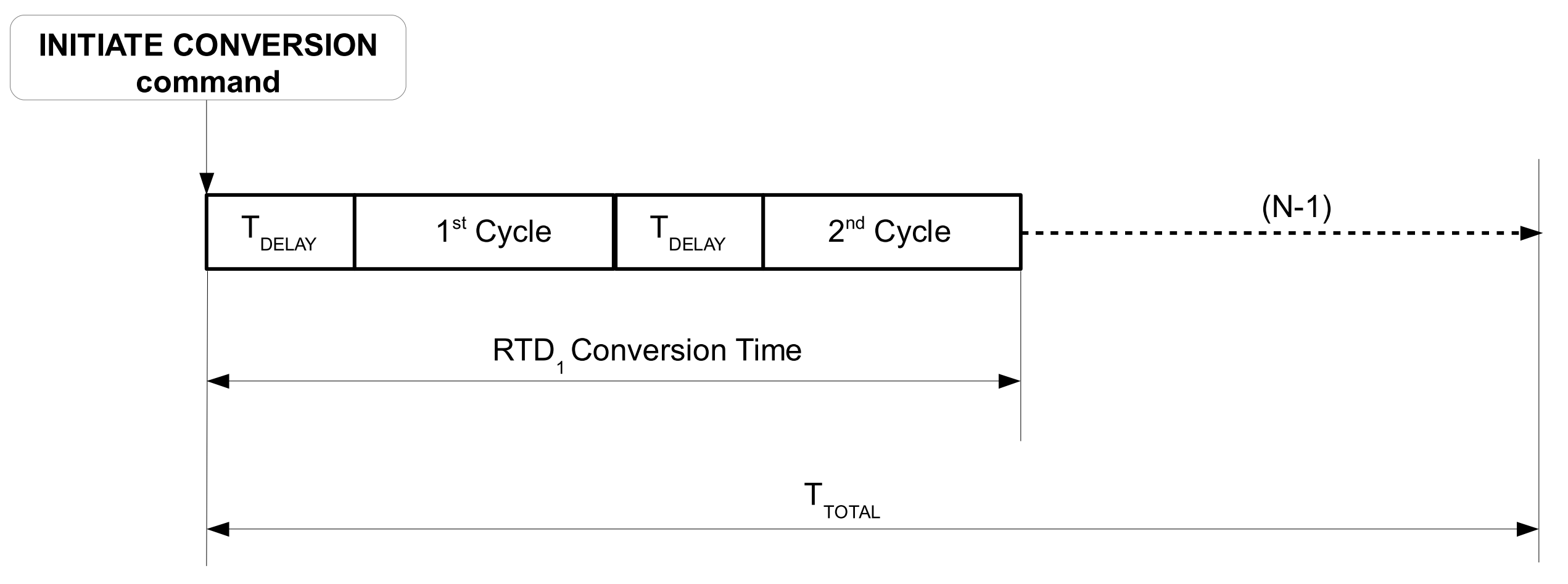# Using the LTC2983 with 18 2-wire RTDs

Introduction

A number of times I have come across system definitions calling for use of a large number of temperature probes. Large number is in the eye of the beholder, of course, but it still brings up a question of how many sensors can interface to the LTC2983, a device that after all boasts 20 analog inputs. Well, let's see... If the thermocouples that are the sensor of interest, then the best that a single LTC2983 can do is 19 thermocouples with one Cold Junction Compensation sensor (diode): each thermocouple occupies one input channel, and one channel is assigned to the diode temp sensor. Such system is a simple expansion of examples described in the datasheet.

How about RTDs? How many of these sensors can be serviced by a single LTC2983? If the goal is to maximize the number of sensors, then sensors with the fewest leads are of interest: a 2-wire RTD is the most likely choice. And just by looking at the examples in the datasheet, it is apparent that 9 RTDs are the most that can connect to the LTC2983. Actually, one can do better than that.

As it turns out, a single LTC2983 temperature measurement device can support up to 18 2-wire RTD probes, as shown in Figure 1. The difference from the datasheet examples is the fact that adjacent RTDs share a node. This in no way compromises the principle of the ratiometric measurement. As usual each RTD measurement consists of simultaneous sensing of two voltages developed across RSENSE and the RTD probe RTDx due to the current IS. Each voltage is sensed differentially, and given the LTC2983’s high common mode rejection ratio, the fact that each RTDs is at a different common mode voltage does not adversely affect the individual measurements.

The choice of the RTD probe depends on the system accuracy and sensitivity requirements. For example, given the fact that 2-wire probes are used, the PT-1000 may prove more robust in the presence of wiring’s parasitic resistance.

Once the RTDs are selected, IS and RSENSE should be chosen so that voltage at the top of the resistor stack (V at the CH1 input) does not exceed the input common mode limit of the LTC2983, over the operating temperature range of the system. This requirement is expressed as follows:

Consider the system shown in Figure 1 and assume the following constraints: 5V supply rail, all RTD probes are PT-100, and the maximum expected temperature measurement is at 150°C. Table 1 shows the channel assignment word for each one of the PT-100 probes. Consult the “Channel Assignment Memory Map” in the LTC2983 data sheet. Note that in this example CH3 senses the RTD1 probe, CH4 senses RTD2, etc.

 Function Bit Field Value Description Sensor Type 31:27 01100 PT-100 Sense Resistor Channel Pointer 26:22 00010 CH2 Sensor Configuration 21:18 0001 2-wire Excitation Current 17:14 1000 1mA RTD Standard 13:12 01 American Curve Custom RTD Data Pointer Address 11:6 000000 NA Length 5:0 000000 NA

The sense resistor, connected to CH2, is configured as shown in Table 2:

 Function Bit Field Value Description Sensor Type 31:27 11101 Sense Resistor (29) Sense Resistor Value Integer 26:10 000000 1111101000 1kΩ Fraction 9:0 0000000000Figure 1. LTC2983 with 18 RTD sensors

RTD Stack Settling Time

Once the excitation current source is enabled, it takes a finite amount of time for the R and C chain to settle, namely the settling time, TS. TS is dependent on the number and value of the individual RS (RSENSE and RTDs), and Cs (capacitance at each input node). The upper bound on TS can be estimated by lumping the total RC, but that yields an overly pessimistic result. Another method to obtain TS is to simply simulate a circuit as shown in Figure 2:Figure 2. Delay Line model of the RTD stack

The results of simulation are shown in Figure 3. Here all Cs were chosen to be 100nF, and RSENSE is 1k. Each line represents TS to within 0.1% of the final value, as a function of a number of RTDs in the stack. For each graph all RTDs are of the same kind.Figure 3. Simulated settling time of the RTD stack

The LTC2983, by default, inserts a delay time TDELAY = 1ms between enabling the excitation source and beginning of the ADC conversion. This, however, is insufficient for any more than two PT-100 probes in the RTD stack, see Figure 3.

The TDELAY may be increased by setting the value in the MUX configuration register, 0x0FF. By default the register is cleared. Each lsb added to the register value represents 100µs added to default TDELAY. Consult the “Supplemental Information” section in the data sheet for more detail on the MUX delay. For example writing 0x10 into 0x0FF results in:

Note that the maximum value of programmable delay is 26.5ms, which is sufficient for settling of at most six PT-1000 devices, given the C = 100nF. See Figures 3 and 4.

The TDELAY is inserted prior to each individual ADC cycle. Each RTD measurement consists of two ADC cycles. Therefore the total conversion time of the stack of RTDs is approximately:

Where TDELAY is programmable by the user, TCONVERT is given in the “Complete System Electrical Characteristics” table in the data sheet, typically 164ms including the default MUX delay, and N is the number of RTDs to be measured. TTOTAL is summarized in Figure 4:Figure 4. Total conversion time of the RTD stack

Conclusion

The LTC2983 can interface to as many as 18 2-wire RTD probes, but be sure to take into account the settling delay is incurred by RC systems—the issue may be exacerbated by the number and type of RTD probes used. The delay issues can be examined using the model and simulation presented here.